# Domain and range of a function#### Everything You Need in One Place

Homework problems? Exam preparation? Trying to grasp a concept or just brushing up the basics? Our extensive help & practice library have got you covered.#### Learn and Practice With Ease

Our proven video lessons ease you through problems quickly, and you get tonnes of friendly practice on questions that trip students up on tests and finals.#### Instant and Unlimited Help

Our personalized learning platform enables you to instantly find the exact walkthrough to your specific type of question. Activate unlimited help now!##### Intros
###### Lessons
1. What is domain and range?
##### Examples
###### Lessons
1. State the domain, range, and whether each is a function

1.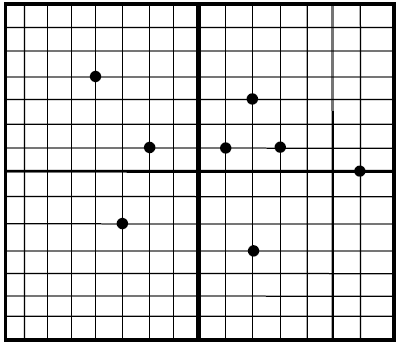2.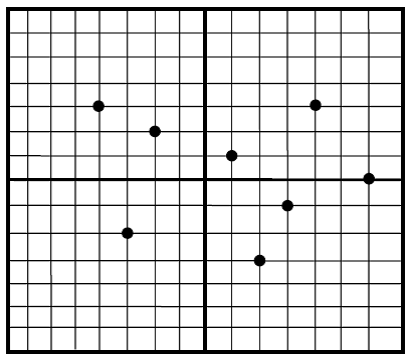3.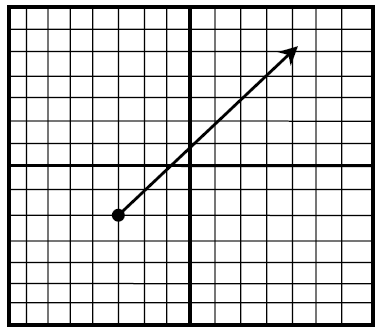4.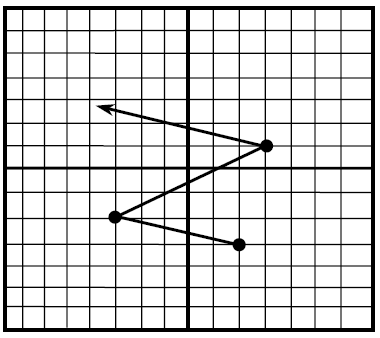5.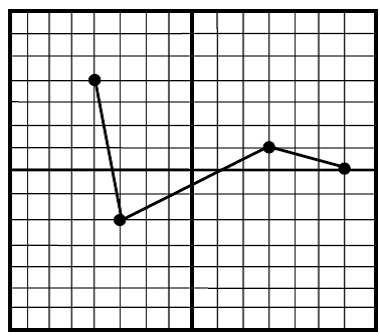6.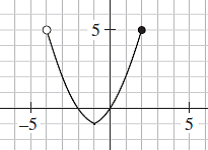(Using the interval notation)

7.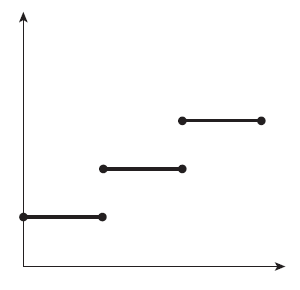(Omit Domain & Range)
8. $(7,2) (3,5) (4,1) (2,6)$
9. $(-3,4) (2,5) (5,1) (-3,8)$
2. State the domain and range of each relation. Then determine whether each is a function.
1.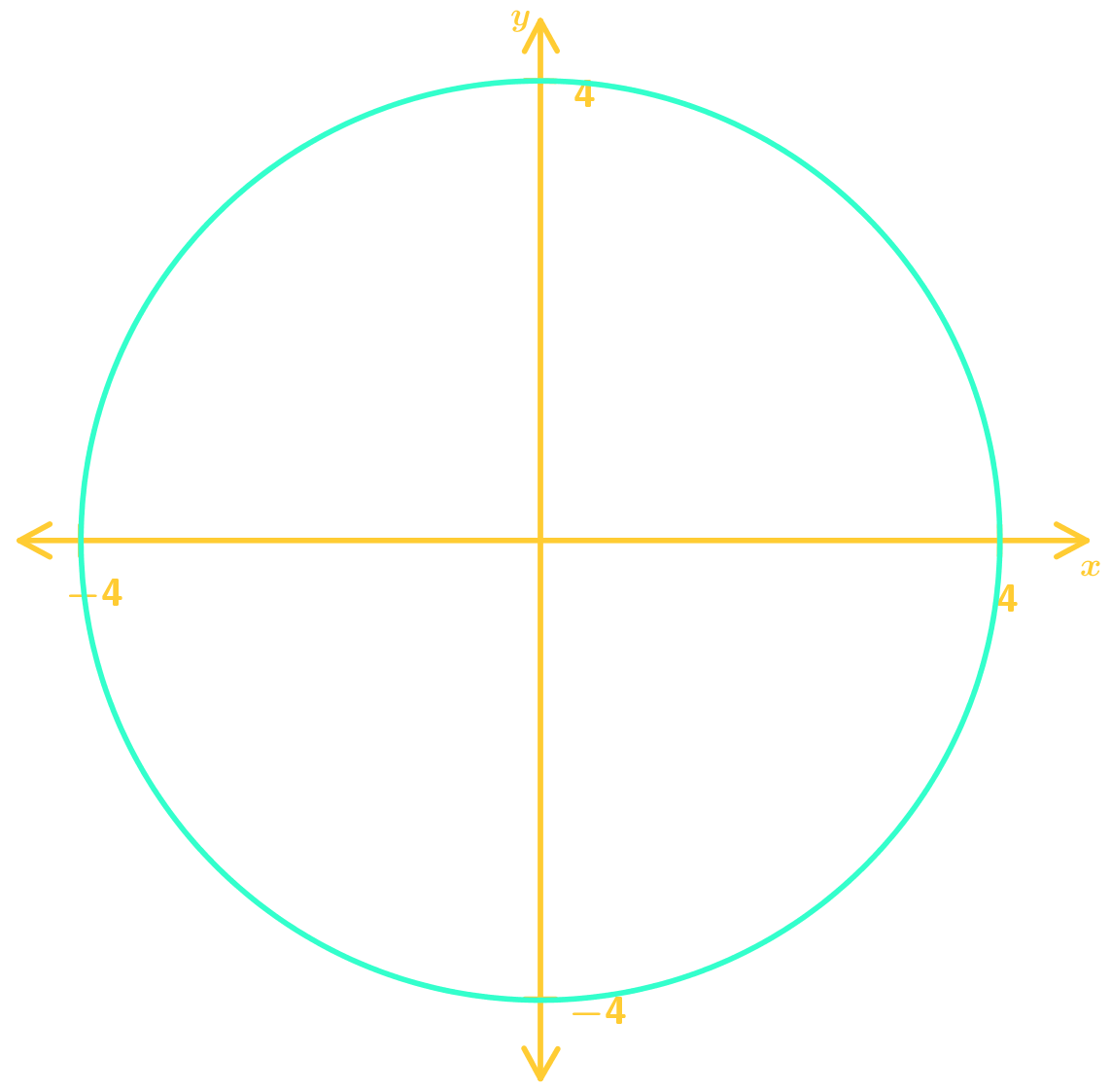2.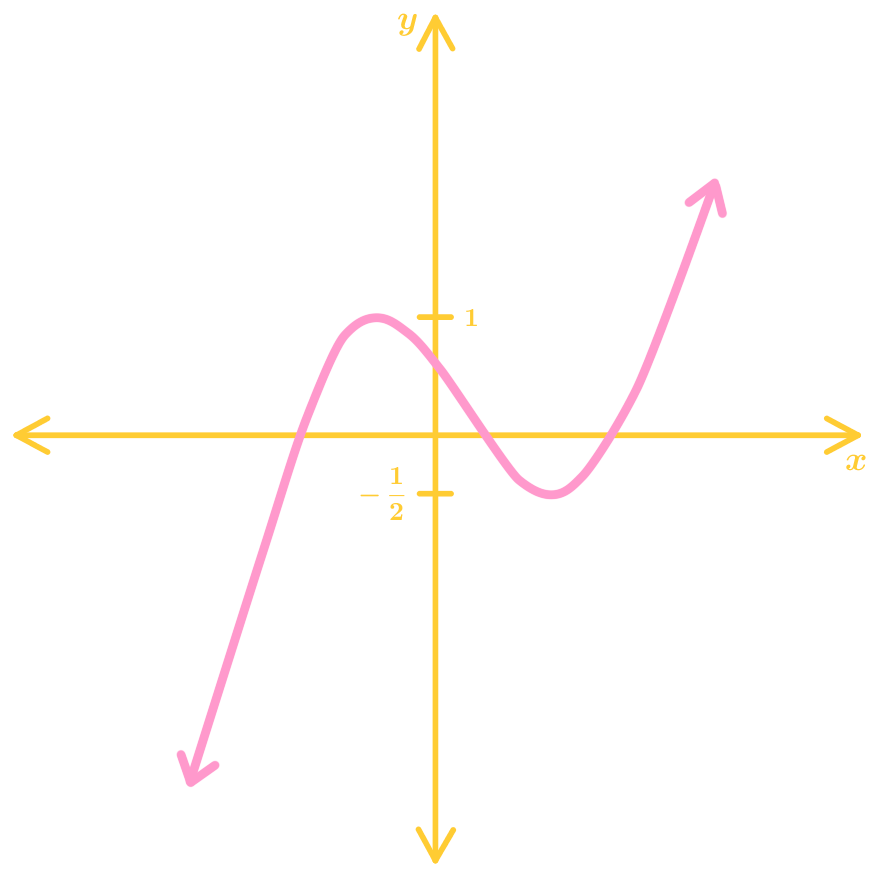3.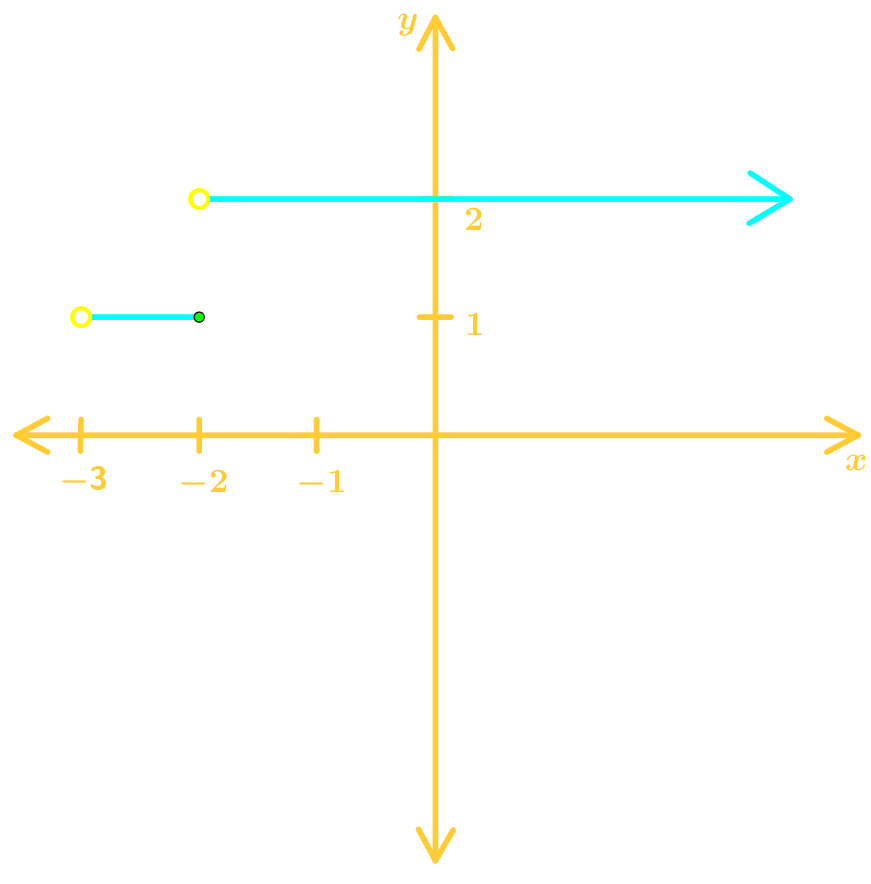4.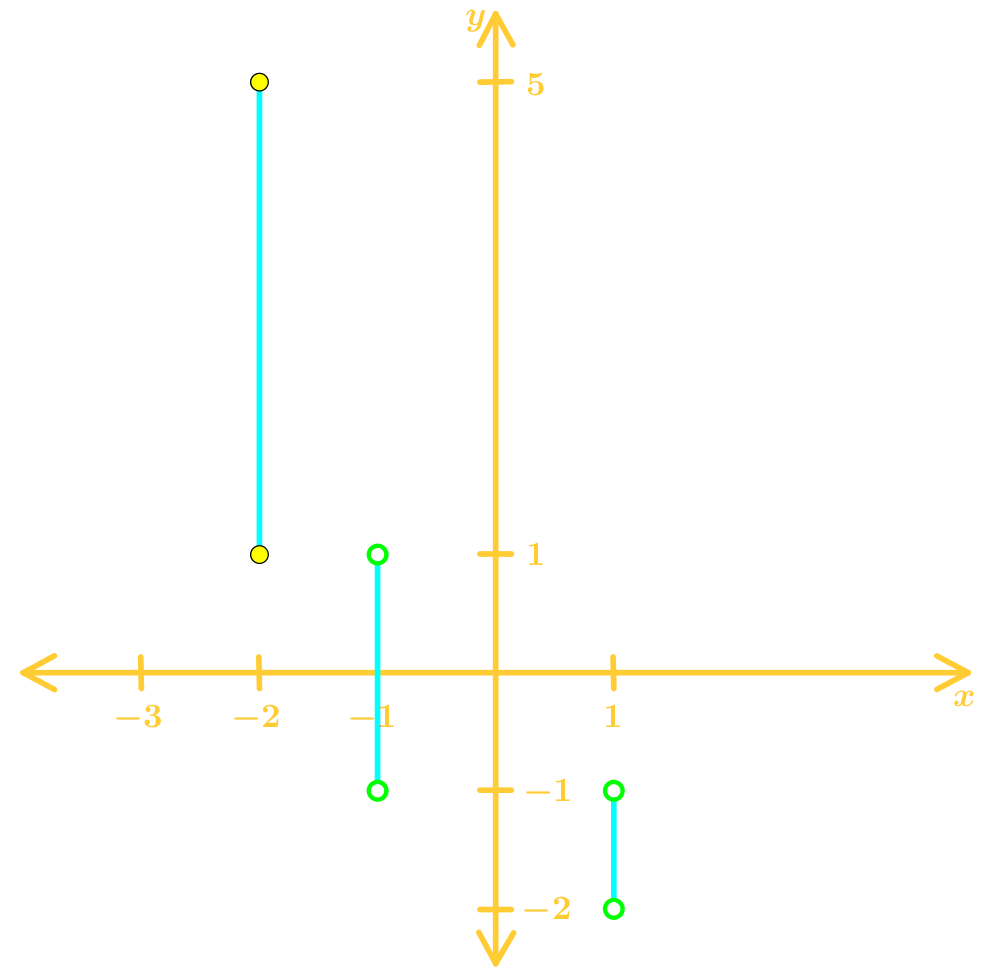5.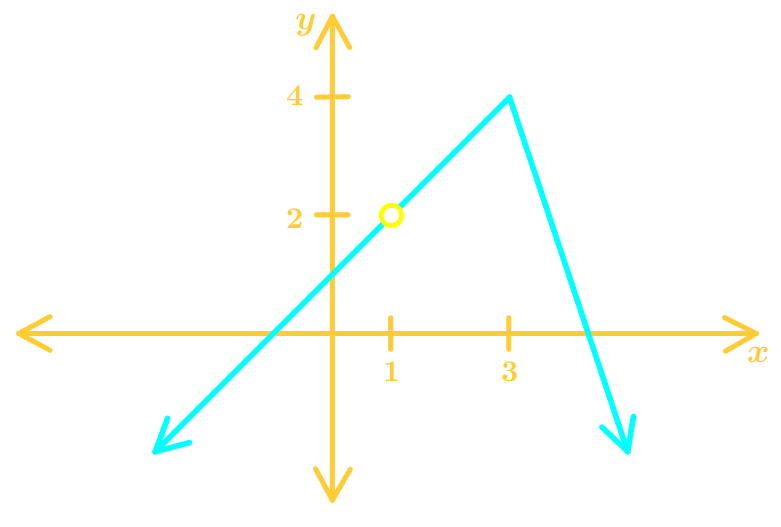6.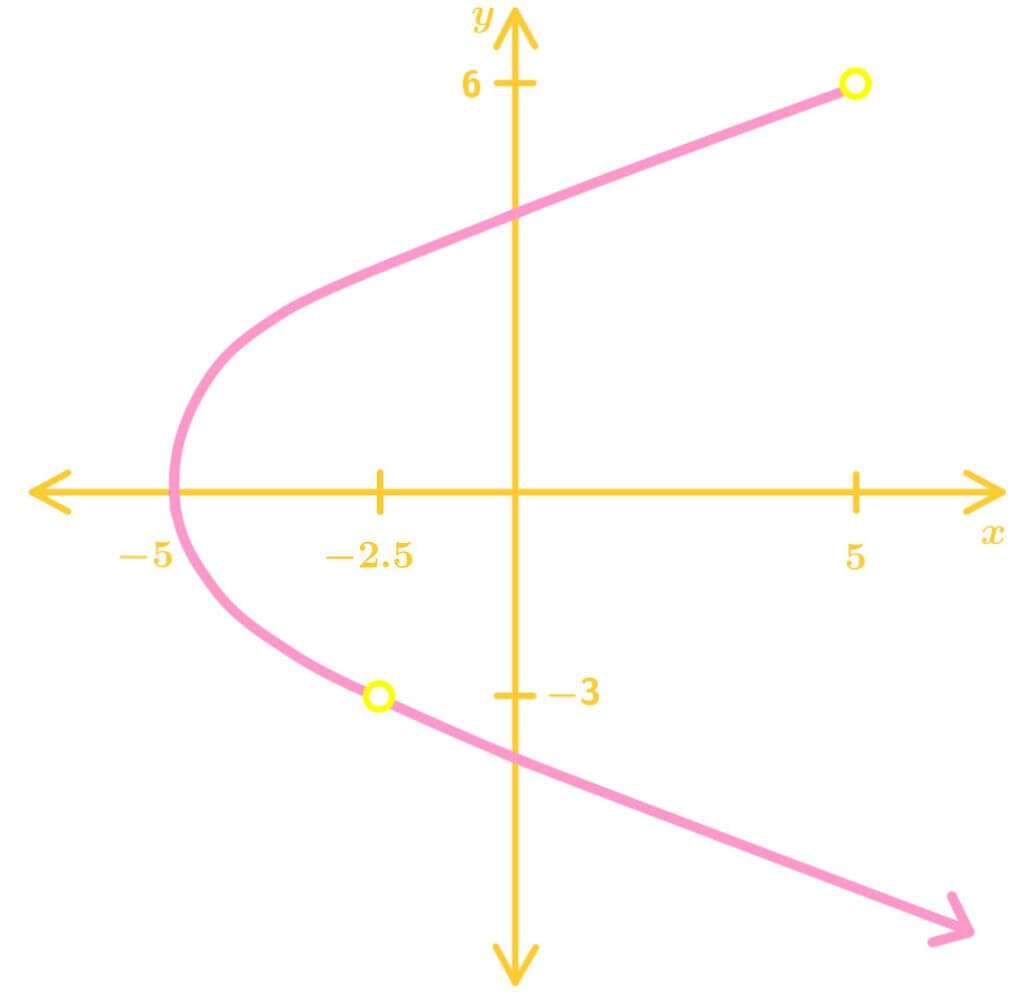3. Sketch a graph of a nonlinear function with the following properties:
i) Domain $x$ $\in$ $I\!R$
ii) Range $y$ $\leq$ 5, $y$ $\in$ $I\!R$
iii) One $x$-intercept
iv) One $y$-intercept
1. Sketch a graph of a nonlinear relation with the following properties:
i) Domain $x$ $\geq -3$, $x$ $\in$ $I\!R$
ii) Range $y$ $\in$ $I\!R$
iii) One $x$-intercept
iv) One $y$-intercept
###### Topic Notes
The domain of a function is all the possible values of x's of ordered pairs; whereas the range of a function is all the possible values of y's of ordered pairs. You can easily find them by graphing the functions or ordered pairs. Let's see how in this lesson.

## What is domain and range

In algebra, when we deal with points on a graph, you may be asked to find its domain and range. Let's learn what each of these mean.

The domain has to do with the values of x in your function. The domain tells us all the possible values of x (the independent variable) that will output real y-values. Two things to note is that in the function you're looking at, the denominator of a fraction can never be 0 and that if your function has a square root, it must be positive (for now).

When you're looking at range, you're now looking at the values of y. The range is all the possible resulting variables of the dependent variable (which is usually y in a function) after substituting in the domain, which we've learned is all the possible values of x.

## How to find the domain of a function

In order to figure out the domain of a function, you'll have to look at the values that's possible (or that we're allowed to use) for the independent variable. This often means that we cannot have a 0 as a denominator, nor can we have negative values in a square root.

## How to find the range of a function

Now let's move on and learn how to find the range of a function. In order to do this, try substituting in different x-values into the function to see what happens to y. You may find that y always remains positive, or it may be negative at some points. Your main goal is to find the minimum and maximum values of y. The best way to figure out what happens to y is to do a sketch with a few points of x substituted in to see what your function looks like.

## Example problems

Now that you've learned the basics on how to find the domain and range of a graph, try out these practice problems!

Question 1:

State the domain, range, and whether it is a function

Solution:

Look at each point to find the domain. In this case, domain is the x value of each dot. So, from the smallest value to the largest we'll get:

D: [ -4, -3, -2, 1, 2, 3, 4, 6]

Now look for the range. Range is the y value of each dot. So, from the smallest value to the largest:

R: [-3, -2, -1, 0, 1, 2, 3]

Note that there are two dots that land on y=3 on the graph. We only need to write it once in the range.

Lastly, we want to determine whether it is a function. For this, we can do a vertical line test. To do this, imagine we draw a vertical line and move the line across the graph. If there is only one point/dot that touches the vertical line at a time, we can determine that it is a function. The graph in this question is a function based on the vertical line test. In practice, we can hold a pen vertically and use it as the vertical line.

Question 2:

State the domain, range, and whether it is a function

Solution:

Domain: $x \leq 3$, OR in interval notation: $(\infty, 3]$

Interval notation helps to show an interval as a pair of numbers. The two numbers represents the endpoints of the interval. The brackets of an interval notation can tell us information too. When we use square brackets [ ], it shows that we want to include the end value as part of the interval. When we use round brackets ( ) it means that we won't include the end value. That's why there's a square bracket at the end of 3, but not before $\infty$.

Range: $y \geq 3$, OR in interval notation: $[-3, \infty)$

This is not a function because it doesn't pass the vertical line test.

Question 3:

State the domain and range of the relation. Then determine whether it is a function.

Solution:

Domain: {${x| x\epsilon R}$}. This $x\epsilon R$ symbol means $x$ can be all real numbers. Or, in interval notation: $(-\infty, +\infty)$

Range: {${y| y\epsilon R}$}. This $y\epsilon R$ symbol means $y$ can be all real numbers. Or, in interval notation: $(-\infty, +\infty)$

This is a function because it passes the vertical line test.

If you wanted to double check your work, try out this online domain and range calculator!